This site uses cookies. By continuing to use this site you agree to our use of cookies. To find out more, see our Privacy and Cookies policy.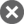#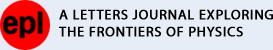## Power-law partition and entropy production of high-energy cosmic rays: Knee-ankle structure of the all-particle spectrum

Roman Tomaschitz

Show affiliations

Roman Tomaschitz 2013 EPL 104 19001
doi:10.1209/0295-5075/104/19001
Received 31 July 2013, accepted for publication 3 October 2013
Published 4 November 2013

### Abstract

A statistical description of the all-particle cosmic-ray spectrum is given in the 1014 eV$10^{14}\ \text{eV}$ to 1020 eV$10^{20}\ \text{eV}$ interval. The high-energy cosmic-ray flux is modeled as an ultra-relativistic multi-component plasma, whose components constitute a mixture of nearly ideal but nonthermal gases of low density and high temperature. Each plasma component is described by an ultra-relativistic power-law density manifested as spectral peak in the wideband fit. The "knee" and "ankle" features of the high– and ultra-high–energy spectrum turn out to be the global and local extrema of the double-logarithmic E3-scaled flux representation in which the spectral fit is performed. The all-particle spectrum is covered by recent data sets from several air shower arrays, and can be modeled as three-component plasma in the indicated energy range extending over six decades. The temperature, specific number density, internal energy and entropy of each plasma component are extracted from the partial fluxes in the broadband fit. The grand partition function and the extensive entropy functional of a non-equilibrated gas mixture with power-law components are derived in phase space by ensemble averaging.

### Introduction

The high–and ultra-high–energy cosmic-ray flux (1014-1020 eV$10^{14}\text{-}10^{20}\ \text{eV}$)  has been measured by air shower arrays such as Tibet-III , KASCADE , KASCADE-Grande , IceCube , HiRes-I and II , the Telescope Array [7,8] and the Pierre Auger Observatory , as well as by the HEGRA , AKENO , GAMMA , Yakutsk  and AGASA  arrays. Here, the goal is to give a statistical description of the broadband spectrum by means of classical ultra-relativistic power-law distributions. We fit the all-particle spectrum with the flux density of a dilute three-component plasma (non-equilibrated, with components at different temperature) and locate the "knees" and "ankles" [1,1517] of the spectrum. The knees are identified as the peaks of the power-law distributions of the plasma components, and the ankles turn out to be the minima in the cross-over regimes of the partial fluxes. These extrema clearly emerge in the E3-scaled flux representation employed in the wideband fit.

We sketch the basic thermodynamic formalism of classical ultra-relativistic power-law ensembles and nonthermal mixtures thereof, starting with the spectral number density parametrized with the Lorentz factor. We then define the specific number count and the internal energy of the gas components, as well as their entropy density. After this overview, we discuss the phase-space measure and probability density of nonthermal power-law ensembles, and derive the grand canonical partition function and the extensive entropy functional of multi-component power-law mixtures involving different particle species at different temperature.

We discuss the practical aspects of broadband spectral fitting with flux densities of classical unequilibrated (stationary non-equilibrium) power-law mixtures in the ultra-relativistic regime, and perform the spectral fit of the all-particle cosmic-ray spectrum above 1014 eV$10^{14}\ \text{eV}$. The spectral flux density of a power-law distribution admits a power-law ascent linear in the log-log flux representation, and a curved descending power-law slope. The curvature is caused by the exponential cutoff determined by the temperature parameter of the Boltzmann factor [18,19]. The broadband spectrum of the all-particle mixture consists of overlapping spectral peaks generated by the partial fluxes of the individual plasma components. By choosing a suitable flux representation (double-logarithmic E3F(E)$E^3F(E)$), we find secondary knees and ankles in the all-particle spectrum. We calculate the thermodynamic parameters of each gas component, and estimate the entropy production of high-energy cosmic rays.

↑ Close

### Mixtures of ultra-relativistic gases in stationary non-equilibrium

An ultra-relativistic classical gas mixture composed of nonthermal power-law components is defined by the spectral number density

dρmix(γ)=i=1Mdρi(γ),dρi(γ)=m3isi2π2(c)3eβiγαiGi(γ)γ21γdγ,(1)

parametrized by the Lorentz factor γ. The positive spectral functions Gi(γ)$G_i (\gamma )$ in the denominator of the power-law components dρi(γ)$\text{d}\rho_i (\gamma )$ are normalized as Gi(1)=1$G_i (1)=1$ and are to be determined from a spectral fit. An M-component mixture is composed of particle species (Zi,mi)$(Z_i ,m_i )$, i=1,,M$i=1,\ldots ,M$, where mi is the mass and Zi the (positive or negative) integer charge number (in multiples of the electron charge e > 0) of the particles in the respective component (Zi,mi)$(Z_i ,m_i )$. The mass parameter mi is a shortcut for mi c2, so that E=miγ$E=m_i \gamma$ is the particle energy in an ideal gas mixture. si is the spin multiplicity and βi=mi/(kBTi)$\beta_i =m_i /(k_{\text{B}} T_i )$ the dimensionless temperature parameter, with Boltzmann constant kB$k_{\text{B}}$. The mixture need not be equilibrated, admitting components at different temperature. The densities (1) are based on grand canonical partitions, allowing a fluctuating particle number via the dimensionless real fugacity parameter αi$\alpha_i$, which is related to the chemical potential μi$\mu_i$ of each gas component by αi=βiμi/mi$\alpha_i =-\beta_i \mu_i /m_i$, and zi=eαi$z_i=\text{e}^{-\alpha_i}$ is the fugacity. The ensemble average leading to (1) is discussed in the next section. The spectral function Gi(γ)$G_i (\gamma )$ is a linear combination of two power laws,

Gi(γ)=γδigi(γ)gi(1),gi(γ)=1+aiγσi,(2)

where δi$\delta_i$ and σi$\sigma_i$ are real power-law exponents and the amplitude ai is positive. Spectral functions of this type are thermodynamically stable  and suitable to model wideband spectra . Quantized power-law ensembles with ai=0$a_i =0$ have been studied in [23,24].

The total particle number Nmix=i=1MNi$N_{\text{mix}} =\sum\limits_{i=1}^M {N_i }$ and the internal energy Umix=i=1MUi$U_{\text{mix}} =\sum\limits_{i=1}^M {U_i}$ of a nonthermal gas mixture in a volume V are determined by the partial densities dρi(γ)$\text{d}\rho_i (\gamma)$ in (1),

Ni=Vγcutidρi(γ),Ui=miVγcutiγdρi(γ).(3)

The lower integration boundary is the cutoff Lorentz factor γcuti1$\gamma_i^{\text{cut}}\ge 1$, referring to particles of a given species (Zi,mi)$(Z_i ,m_i )$ with energies exceeding Ecuti=miγcuti$E_i^{\text{cut}} =m_i \gamma _i^{\text{cut}}$, and adjusted to the energy range of the available data sets. The ultra-relativistic limit of the power-law densities dρi(γ)$\text{d}\rho _i (\gamma)$ is obtained by replacing γ21γ$\sqrt{\gamma^2-1} \to \gamma$ in (1) and is applicable if γcuti1$\gamma_i^{\text{cut}} \gg1$. The thermal relativistic Maxwell-Boltzmann distribution is recovered with δi=0$\delta_i =0$, gi(γ)=1$g_i (\gamma )=1$ and γcuti=1$\gamma_i^{\text{cut}} =1$ as lower integration boundary in (3).

The partition function of a gas component (Zi,mi)$(Z_i ,m_i)$ is denoted by Z(Zi,mi)$Z_{(Z_i ,m_i )}$. Since particle number and internal energy are related to the grand partition function by Ni=(logZ(Zi,mi)),αi$N_i =-(\log Z_{(Z_i ,m_i)})_{,\alpha_i}$ and Ui=mi(logZ(Zi,mi)),βi$U_i =-m_i (\log Z_{(Z_i ,m_i )} )_{,\beta_i }$, we can identify the partition function Zmix$Z_{\text{mix}}$ of a nonthermal mixture as logZmix=i=1Mξi$\log Z_{\text{mix}} =\sum\limits_{i=1}^M {\xi_i}$, where ξi=logZ(Zi,mi)$\xi_i =\log Z_{(Z_i,m_i)}$ and

ξi=V4πsim3i(2πc)3eαiγcutieβiγGi(γ)γ21γdγ.(4)

Apparently ξi=Ni$\xi_i =N_i$ holds for this classical distribution, and Zmix$Z_{\text{mix}}$ is the product of the individual components Z(Zi,mi)$Z_{(Z_i ,m_i)}$. Zmix$Z_{\text{mix}}$ depends on the temperature variables βi$\beta_i$ and the fugacity parameters αi$\alpha_i$; the latter can be parametrized by particle number and temperature, αi(βi,Ni/V)$\alpha_i (\beta_i ,N_i /V)$, by solving (4). The entropy of a nonthermal gas mixture is an extensive quantity,

Smix=i=1MSi,Si=kB(logZ(Zi,mi)+βimiUi+αiNi),(5)

obtained from an ensemble average of power-law partitions, see the next section.

↑ Close

### Power-law partitions of multi-component mixtures in phase space

We give a probabilistic derivation of the classical spectral number density dρmix(γ)$\text{d}\rho_{\text{mix}}(\gamma)$ of an ultra-relativistic gas mixture in stationary non-equilibrium, cf. (1). To this end, we consider the phase space of n particles of a gas component (Zi,mi)$(Z_i ,m_i )$ labeled by charge number and mass in an M-component mixture, i=1,,M$i=1,\ldots ,M$. The phase-space measure of component (Zi,mi)$(Z_i ,m_i )$ is

d3n(Zi,mi)(p,q)=1(2π)3nd3p1d3q1d3p2d3q2d3pnd3qn,d3pk=4πm3ic3γ2k1γkdγk,k=1,,n.(6)

The d3qk$\text{d}^3q_k$ integrations range over a volume V, and the dγk$\text{d}\gamma_k$ integrations refer to the interval γcutiγk$\gamma_i^{\text{cut}} \le \gamma_k \le \infty$, cf. after (3), where γcuti$\gamma_i^{\text{cut}}$ is the cutoff Lorentz factor of the respective gas component (Zi,mi)$(Z_i ,m_i )$. The angular integration over the solid angle dΩ$\text{d}\Omega$ has been carried out in (6) and gives the factor 4π$4\pi$. The scale factor (2π)3n$(2\pi \hbar)^{3n}$ renders the measure dimensionless.

### Probability density and grand partition function of mixtures with power-law components

The probability density on the n-particle states of a gas component (Zi,mi)$(Z_i,m_i)$ factorizes as

f(Zi,mi)n(p,q)b(Zi,mi)nh(Zi,mi)n=snin!b(Zi,mi)n(p,q)h(Zi,mi)n(p,q),=exp(ξiβik=1nγkαin),=exp(k=1nlogGi(γk)).(7)

The factor 1/n!$1/n!$ accounts for the indistinguishability within the particle species labeled (Zi,mi)$(Z_i ,m_i )$, and the positive integer si for the spin multiplicity of this component. βi=mi/(kBTi)$\beta_i=m_i/(k_\text{B} T_i )$ is the temperature parameter of the respective gas component, and ξi$\xi_i$ is a normalization constant.

The normalization condition for the phase-space probability of an M-component mixture is

n1,,nM=0i=1Mf(Zi,mi)ni(p,q)d3ni(Zi,mi)(p,q)=i=1Mn=0f(Zi,mi)n(p,q)d3n(Zi,mi)(p,q)=1,(8)

where summation and integration sign can be interchanged, as well as the integration and product signs. The (p,q)$(p,q)$ variables of the density and measure will occasionally be omitted. The integrals in (8) factorize into one-particle states, and normalization is achieved by identifying ξi=logZ(Zi,mi)$\xi_i =\log Z_{(Z_i ,m_i )}$ in (7) with the partition function (4). Thus,

Z(Zi,mi)=n=0snin!exp(βik=1nγkαin)h(Zi,mi)nd3n(Zi,mi),(9)

for each gas component (Zi,mi)$(Z_i ,m_i )$. The partition function of an ideal mixture factorizes, Zmix=i=1MZ(Zi,mi)$Z_{\text{mix}} =\prod\limits_{i=1}^M {Z_{(Z_i ,m_i)}}$, or additively ξmix=logZmix$\xi_{\text{mix}} =\log Z_{\text{mix}}$.

↑ Close

### Entropy of a nonthermal gas mixture

The expectation values of particle number and internal energy of a gas component (Zi,mi)$(Z_i ,m_i)$ read

n=0nf(Zi,mi)n(p,q)d3n(Zi,mi)(p,q)=dξidαi=n(Zi,mi)=Ni,(10)

n=0(mik=1nγk)f(Zi,mi)n(p,q)d3n(Zi,mi)(p,q)=midξidβi=u(Zi,mi)=Ui,(11)

where u=mik=1nγk$u=m_i \sum\limits_{k=1}^n{\gamma_k}$, and Ni=ξi$N_i =\xi_i$, cf. (4). The spectral number density (1) follows from the phase-space average (10). The total particle count Nmix$N_{\text{mix}}$ and internal energy Umix$U_{\text{mix}}$ are obtained by summing over the particle species, cf. (3). The total entropy Smix=i=1MSi$S_{\text{mix}} =\sum\limits_{i=1}^M {S_i}$ of the mixture is found by adding the partial entropies defined by the average

Si=kBn=0snin!h(Zi,mi)nb(Zi,mi)nlogb(Zi,mi)nd3n(Zi,mi),(12)

which is the expectation value s(Zi,mi)$\langle s\rangle_{(Z_i ,m_i )}$ of the phase-space random variable s=kB(ξi+βik=1nγk+αin)$s=k_\text{B} (\xi_i +\beta_i \sum\limits_{k=1}^n {\gamma_k } +\alpha_i n)$,

Si=kBn=0(ξi+βik=1nγk+αin)f(Zi,mi)nd3n(Zi,mi).(13)

The partial entropies Si stated in (5) are recovered from (13) by substitution of the averages (10) and (11).

↑ Close
↑ Close

### Spectral flux densities of nonthermal mixtures

We write the components of the total spectral number density dρmix$\text{d}\rho_{\text{mix}}$ in (1) as

dρi(E)dE=4πm2isieαi(2πc)3gi(1)gi(γ)γ1δieβiγγ21,(14)

with spectral function gi(γ)$g_i (\gamma)$ in (2), and replace the Lorentz factor by the particle energy γ=E/mi$\gamma =E/m_i$. The mass mi stands for mi c2, si is the spin degeneracy of component (Zi,mi)$(Z_i ,m_i )$, cf. after (1), and zi=eαi$z_i =\text{e}^{-\alpha_i}$ the fugacity. βi=mi/(kBTi)$\beta_i =m_i/(k_\text{B}T_i)$, and δi$\delta_i$ is a real power-law exponent. The amplitude of the second power law in gi(γ)=1+aiγσi$g_i (\gamma )=1+a_i \gamma^{\sigma_i}$ is positive, ai0$a_i \ge 0$, and we will use positive exponents σi$\sigma_i$. Hence,

dρi(E)dE=4πsieαˆi(2πc)3E2δieβˆiE1+aˆiEσi1m2iE2,(15)

where we introduced the shortcuts βˆi=1/(kBTi)$\hat{\beta}_i =1/(k_\text{B}T_i)$, aˆi=ai/mσii$\hat{a}_i =a_i /m_i^{\sigma_i}$ and

αˆi=αilog(1+ai)δilogmi.(16)

We also note eαˆi=zimδii(1+ai)$\text{e}^{-\hat{\alpha}_i}=z_im_i^{\delta_i}(1+a_i)$. We will use dimensionless quantities: E and mi in eV units, and the units chosen for length, time and temperature are, respectively, meter, second and kelvin.

The spectral flux density of a gas component is found by multiplying the number density (15) with the particle velocity υi=c1m2i/E2$\upsilon_i =c\sqrt{1-m_i^2 /E^2}$,

Fi(E)=c4π1m2iE2dρidE=csieαˆi(2πc)3E2δieβˆiE1+aˆiEσi(1m2iE2).(17)

Density Fi(E)$F_i (E)$ is the number flux density per steradian; the energy flux is obtained by rescaling Fi(E)$F_i (E)$ with E. Wideband spectra are assembled by adding the partial flux densities of the gas components, Fmix(E)=i=1MFi(E)$F_{\text{mix}} (E)=\sum\limits_{i=1}^M {F_i(E)}$. To locate the "knees" and "ankles" of the spectrum in log-log plots, it is convenient to rescale Fmix(E)$F_{\text{mix}}(E)$ with a suitable power Ek. The rescaled density EkFmix(E)$E^kF_{\text{mix}} (E)$ is thus obtained by adding the partial fluxes

EkFi(E)[ eVk1m2sr1s1]=yˆiE2+kδieβˆiE1+aˆiEσi(1m2iE2),(18)

where we defined the amplitude

yˆi=csieαˆi(2πc)30.3146×1027si2eαˆi,(19)

using dimensionless quantities, cf. after (16). In the ultra-relativistic regime, we can drop the factor 1m2i/E2$1-m_i^2 /E^2$ in (18). Typically, the partial density (18) has a peak defined by an ascending power-law slope E2+kδi$E^{2+k-\delta_i}$ and a descending slope E2+kδiσi$E^{2+k-\delta_i-\sigma_i}$ terminating in exponential decay. The fitting parameters are the amplitudes yˆi$\hat{{y}}_i$, aˆi$\hat{{a}}_i$ and the exponents δi$\delta_i$, σi$\sigma_i$ determining location, height and the two power-law slopes of each peak, as well as the temperature parameter βˆi$\hat{\beta}_i$ defining the exponential cutoff.

The chemical potential μi=αi/βˆi$\mu_i =-\alpha_i /\hat{\beta}_i$, temperature and fugacity of each gas component can be extracted from the fitting parameters, cf. (16) and (19):

zi=eαˆimδii(1+aˆimσii),eαˆi3.18×10272siyˆi,(20)

and μi[eV]=kBTilogzi$\mu_i \text{[eV]}=k_\text{B} T_i \log z_i$, with kBTi=1/βˆi$k_\text{B} T_i =1/\hat{\beta}_i$. All quantities are measured in units stated after (16). The specific particle density Ni/V$N_i /V$ in (3) reads

NiV[m3]=4πcyˆiEcutiE2δieβˆiE1+aˆiEσi1m2iE2dE,(21)

where the lower energy cutoff (integration boundary) is related to the Lorentz factor by Ecuti=miγcuti$E_i^{\text{cut}} =m_i \gamma_i^{\text{cut}}$. The energy density Ui/V[eV m3]$U_i/V[\text{eV m}^{-3}]$, cf. (3), is obtained by adding a factor E to the integrand in (21). The specific densities Ni/V$N_i/V$ and Ui/V$U_i /V$ become independent of the particle mass mi in the ultra-relativistic regime m2i/Ecut2i1$m_i^2 /E_i^{\text{cut}2} \ll 1$, where the root in the integrand (21) can be dropped, so that the mass is absorbed in the fitting parameters. The partial entropies (5) are assembled from the specific number and energy densities,

1kBSiV[m3]=(1logzi)NiV+1kBTiUiV,(22)

where we have put αi=logzi$\alpha_i =-\log z_i$ in (5). Fugacity and temperature are extracted by way of (20). In the ultra-relativistic limit, we do not need to know the mass of the particles to calculate the specific number and energy densities (21) from the spectral fit. However, mass enters the entropy density through the fugacity, cf. table 1.

Table 1:.  Thermodynamic parameters of the ultra-relativistic number densities dρi/dE$\text{d}\rho_i/\text{d}E$, cf. (15), generating the flux components Fi=1,2,3(E)$F_{i=1,2,3} (E)$ of the all-particle spectrum in fig. 1. Ni/V$N_i/V$ is the specific number density and Ui/V$U_i /V$ the energy density of the respective plasma component, calculated via (21) with lower cutoff energy Ecuti1014 eV$E_i^{\text{cut}} \approx 10^{14}\ \text{eV}$. Recorded are temperature Ti, fugacity zi and chemical potential μi$\mu_i$, cf. (20), as well as the partial entropy densities Si/V$S_i /V$, cf. (22). The fourth row lists the total specific densities Nmix/V$N_{\text{mix}} /V$, Umix/V$U_{\text{mix}} /V$ and Smix/V$S_{\text{mix}} /V$ of the gas mixture, cf. (3) and (5). The entropy production is mainly due to the fugacity term in (22), and varies only weakly with particle mass, since the fugacities logarithmically enter the partial entropies. Fugacity, chemical potential and entropy are listed for a proton gas with mp938.27×106 eV$m_p \approx 938.27\times 10^{6}\ \text{eV}$. Helium nuclei with mass mHe3.97mp$m_{\text{He}} \approx 3.97m_p$ produce an entropy density of SHemix/(kBV)3.8×1010$S_{\text{mix}}^{\text{He}}/(k_\text{B} V)\approx 3.8\times 10^{-10}$, and nickel nuclei with mNi58.7mp$m_{\text{Ni}} \approx 58.7m_p$ produce SNimix/(kBV)4.2×1010$S_{\text{mix}}^{\text{Ni}} /(k_\text{B} V)\approx 4.2\times 10^{-10}$, so that the actual mass composition, which is not yet known in the energy range of fig. 1, cannot significantly change the recorded protonic entropy estimates. At 10 GeV, the He/H abundance ratio is 0.048, and the Ni/H ratio is about 2×104$2\times 10^{-4}$ .

 i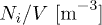Ni/V [m−3]$N_i /V~\text{[m}^{-3}]$Ui/V [eV m−3]$U_i /V~\text{[eV m}^{-3}\,]$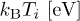kBTi [eV]$k_\text{B} T_i~\text{[eV]}$logzi$\log z_i$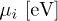μi [eV]$\mu_i~\text{[eV]}$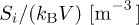Si/(kBV) [m−3]$S_i/(k_\text{B} V)~\text{[m}^{-3}\,]$ 13.15×10−12$3.15\times 10^{-12}$ 745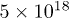5×1018$5\times 10^{18}$ −113−5.6×1020$-5.6\times 10^{20}$3.6×10−10$3.6\times 10^{-10}$ 21.25×10−14$1.25\times 10^{-14}$ 18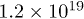1.2×1019$1.2\times 10^{19}$ −131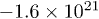−1.6×1021$-1.6\times 10^{21}$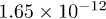1.65×10−12$1.65\times 10^{-12}$ 3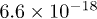6.6×10−18$6.6\times 10^{-18}$ 0.031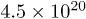4.5×1020$4.5\times 10^{20}$ −136.5−6.2×1022$-6.2\times 10^{22}$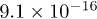9.1×10−16$9.1\times 10^{-16}$ mix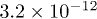3.2×10−12$3.2\times 10^{-12}$ 763 – – –3.6×10−10$3.6\times 10^{-10}$
↑ Close

### The multi-component plasma of high-energy cosmic rays

The fit of the all-particle cosmic-ray spectrum in fig. 1 is performed with the ultra-relativistic limit of the flux density Fmix(E)$F_{\text{mix}} (E)$, cf. (18). We scale this density with E3, defining fmix=E3Fmix(E)$f_{\text{mix}} =E^3F_{\text{mix}}(E)$, so that

fmix(E)=i=1Mfi,fi(E)yˆiE5δieβˆiE1+aˆiEσi.(23)

The fitting parameters in (23) are the power-law exponents δi$\delta_i$, σi$\sigma_i$, the amplitudes yˆi$\hat{{y}}_i$, aˆi$\hat{{a}}_i$, and the temperature parameters βˆi=1/(kBT